## Income Tax Formula

In this post, we’ll examine a couple of ideas for computing income tax in Excel using tax tables. Specifically, we’ll use VLOOKUP with a helper column, we’ll remove the helper column with SUMPRODUCT, and then we’ll use data validation and the INDIRECT function to make it easy to pick the desired tax table, such as single or married filing joint.

## Objective

Before we get ahead of ourselves, let’s be clear about our objective. We want to store a tax table in Excel. We want to enter a taxable income and have Excel compute the tax amount, the marginal tax rate, and the effective tax rate. This idea is illustrated in the screenshot below.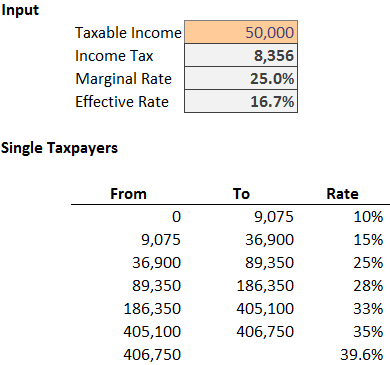Since this is Excel, there are many ways to achieve the goal. In this post, we’ll use VLOOKUP with a helper column, we’ll then eliminate the helper column with SUMPRODUCT, and then we’ll allow the user to select the correct tax table with INDIRECT. Let’s explore each approach.

## VLOOKUP

The VLOOKUP function is a traditional lookup function that returns a related value from a lookup table.

Since the VLOOKUP function returns a single value, and not an aggregated sum of many values, we’ll need to modify our tax table by adding a helper column. The helper column will compute the cumulative tax for each tax bracket, as shown below.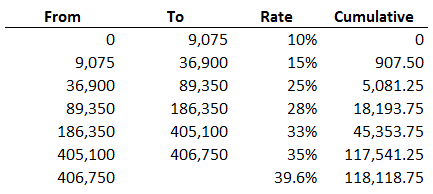The cumulative helper column formula is straightforward, we simply apply the marginal rate to the bracket income. The sample file below contains the formula for reference.

If we assume a taxable income of \$50,000, we need to write a formula that basically performs the following math:

`=5081.25+((50000-36900)*.25)`

We can use VLOOKUP to obtain all of the related values from the tax table based on the taxable income. The basic syntax of the VLOOKUP function follows:

`VLOOKUP(lookup_value, table_array, col_index_num, [range_lookup])`

Where:

• lookup_value is the value we are seeking
• table_array is where we are looking
• col_index_num is the column that has the value to return
• [range_lookup] will be TRUE since we are performing a range lookup, not seeking an exact matching value

Let’s apply the VLOOKUP to the tax calculator below.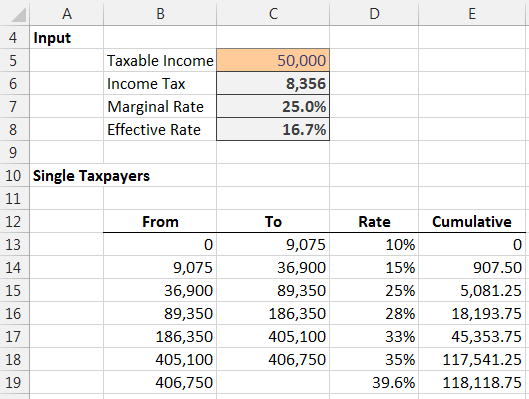We’ll substitute each table value in the following formula with a VLOOKUP function:

`=5081.25+((50000-36900)*.25)`

Doing so produces the following formula:

`=VLOOKUP(C5,B13:E19,4,TRUE)+(C5-VLOOKUP(C5,B13:E19,1,TRUE))*VLOOKUP(C5,B13:E19,3,TRUE)`

Now, we can enter a taxable income into C5 and our formula applies the desired math.

But, what if we did not want our worksheet to contain the cumulative tax amount helper column? We can use the SUMPRODUCT function instead.

## SUMPRODUCT

The SUMPRODUCT function is able to return the sum of multiple products. That is, it is able to apply the rate to each bracket and return the sum. This capability eliminates the need for a helper column.

This function is trickier to visualize because it operates on multiple cells at once.

For our discussion, let’s consider the updated calculator in the screenshot below, which contains no helper column.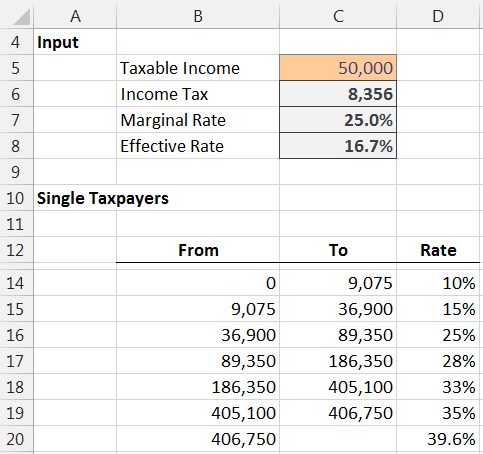If the taxable income was \$50,000, we would like Excel to perform the following math. It needs to multiply all \$50,000 by 10% because all \$50,000 is taxed by at least 10%. To that, we need Excel to add 5% of 40,925 (50,000-9,075), because the differential rate of the next bracket is 5% (15%-10%). To that, we need to add 10% of 13,100 (50,000-36,900), because the differential rate of that bracket is 10% (25%-15%).

The differential rate idea is illustrated in the screenshot below.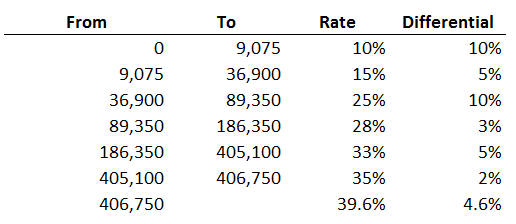Using the differential rate instead of the marginal rate simplifies our SUMPRODUCT function.

To visualize the math, we could set up a new column that shows the amount to be applied to each differential rate by subtracting \$50,000 from each income bracket, as shown below.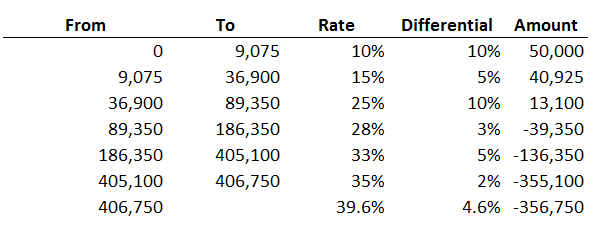To see the extended tax, let’s add another column that computes the amount by the differential rate, shown below.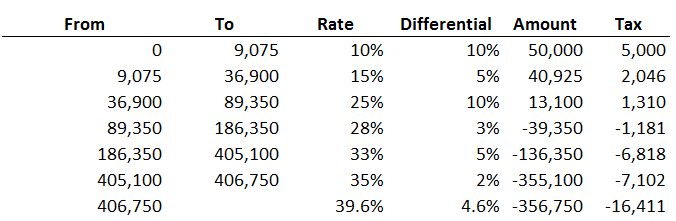But, we only want to include the tax rows that are positive numbers. We could add another column that converts the negative values to zero, and then add it up, as shown below.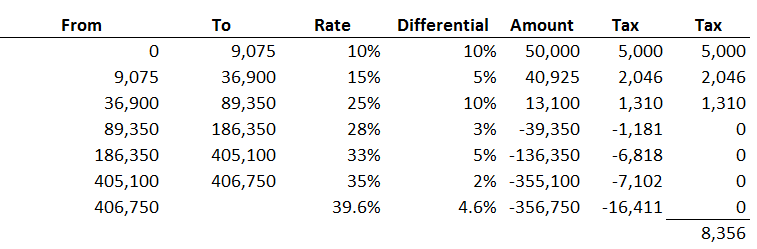Fortunately, \$8,356 is the same value returned by our VLOOKUP formula, so, I think our math is good.

With the basic idea in mind, let’s reconsider the following worksheet.Since our goal is to eliminate helper columns, we can ask the SUMPRODUCT function to compute the differential rate, compute the amount to apply to each rate, and exclude negative values all in a single function:

`=SUMPRODUCT(D14:D20-D13:D19,C5-B14:B20,N(C5>B14:B20))`

The SUMPRODUCT function returns the sum of the product of its arguments. That is, it multiplies its arguments together, and then computes the sum. Let’s walk through the arguments. The first argument, D14:D20-D13:D19, computes the differential rate. (Note that D13 needs to be an empty cell, not a text string, which is why we made row 13 skinny.) The second argument, C5-B14:B20, computes the amount to apply to each differential rate. The third argument, N(C5>B14:B20), excludes negative values by converting the boolean result of the comparison, eg, TRUE or FALSE, to 1 or 0. When Excel multiplies the row by 0 it is excluded from the sum.

#### Illustration

I’ll set up some new formulas in another area of the worksheet to illustrate the math that is performed by SUMPRODUCT. Note that the cell references below are different than the ones above. SUMPRODUCT uses columns B and C. To illustrate the logic, I set up column D to represent the first argument (differential rate), column E to represent the second argument (amount), and column F to represent the third argument (TRUE/FALSE logic to include or exclude the row). Column G multiplies these columns, and G33 provides the sum.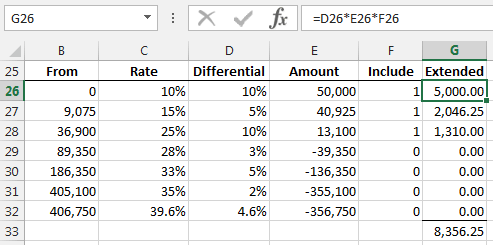If your goal is to eliminate helper formulas, SUMPRODUCT can be helpful. However, my preferred approach is to use VLOOKUP and INDIRECT as demonstrated next.

## VLOOKUP and INDIRECT

We want to provide the user with the ability to select a filing status, such as single or MFJ. We’ll store rates for each filing status in a separate Excel table (Insert > Table). We’ll name each table with the desired filing status. We’ll use data validation to provide an in-cell drop down with the list of choices. We’ll use the VLOOKUP approach demonstrated above to retrieve related values and compute the taxable income. We’ll use the INDIRECT function to tell Excel which table to use for the lookup. Whew…that’s a lot of moving parts. Since they have been explored previously in this blog, rather than provide each of the steps here, I’ll simply provide references to the related blog posts in the additional resources list below.

Here is our final product: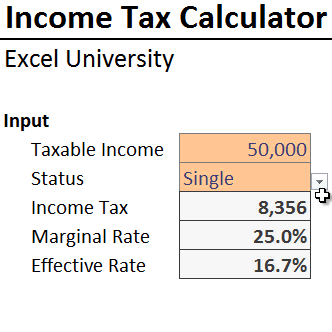All approaches discussed above are included in the sample file below. Please feel free to check out the file as needed.

If you have any alternative approaches, please share by posting a comment below…thanks!### Jeff Lenning

I love sharing the things I've learned about Excel, and I built Excel University to help me do that. My motto is: Learn Excel. Work Faster.

### Excel is not what it used to be.

You need the Excel Proficiency Roadmap now. Includes 6 steps for a successful journey, 3 things to avoid, and weekly Excel tips.

### Want to learn Excel?

Access all Undergrad and Masters lessons with a Campus Pass or CPE Pass. Includes on-demand training plus live office hours.

1.Don Steele on January 21, 2015 at 1:05 pm

Good job, Lenning. I have prepared a workbook that includes the Canada tax regime for personal tax as well as the USA ones and other analyses. If you are interested, how could I send you the XLSX file so everyone can benefit?

•jefflenning on January 21, 2015 at 2:06 pm

Don…excellent! I’ll email you and if you email the file back to me I’ll post it here for others…thanks for sharing!
Thanks
Jeff

2.Sal on July 2, 2015 at 8:20 am

Can you email me the excel spreadsheet?

3.Jeremy Kim on July 27, 2015 at 12:54 pm

I checked the calculations for Don’s workbook for the Canadian side of things. Unfortunately it is slightly inaccurate since he didn’t account for “Personal Amount” for income tax.

I don’t know if US income tax has this but in Canadian income tax, we all get a base “Personal Amount” that we do not get taxed on. In 2015, this amount is \$11327 for federal income tax and varying amounts for provincial income taxes depending on which province you live in.

I created a workbook that automatically calculates this for Federal, BC and Ontario using the vlookup method. My numbers have been verified within <\$1~\$4 (at the millionaire end) accuracy with two other income tax calculators found online (simpletax and EY).

One thing I found confusing though, was that my BC and Federal numbers are consistently accurate from the lowest to highest incomes but once my Ontario numbers go beyond the \$50K income range, they start to go off by huge magnitudes (from hundreds to tens of thousands of dollars in tax payable). I verified the basic data (ranges, %ages, etc.) but I believe I have no errors there.

If there are any other Canadians reading this, do any of you know if there are any other variables specific to Ontario income tax that I've perhaps missed at the upper income ranges?

•jefflenning on August 10, 2015 at 8:17 am

Thanks for sharing Jeremy! We’ll see if any other Canadians can assist!
Thanks,
Jeff

•Aran Poyntz on November 20, 2015 at 11:34 am

Hi Jeremy,
How did you factor in/out the “Personal Amount” on the federal taxes, all my calculations error out?

regards,

•DMurray on May 31, 2017 at 12:17 pm

@ Jeremy Kim
Would you be willing to post your spreadsheet? Let me know. Thanks!

4.Valerie on March 15, 2016 at 6:19 pm

Just wanted to write and say thanks for the guidance!

5.arider on April 23, 2016 at 7:34 pm

Thanks for the explanation of SUMPRODUCT! This is the function I have been looking for during all these long years of tax-paying!

6.Rob Mahn on April 3, 2017 at 8:32 am

The SUMPRODUCT calculation is using the wrong table. Is should be using the TO amounts instead of the FROM amounts. This caused an error of tax on 1 dollar as it crosses each tax bracket.

=SUMPRODUCT(D14:D20-D13:D19,C5-B14:B20,N(C5>B14:B20))

should be

=SUMPRODUCT(D14:D20-D13:D19,C5-C13:C19,N(C5>C13:C19))

•Jeff Lenning on April 3, 2017 at 4:46 pm

Thanks for the assist, really appreciate it!

7.Thu on September 22, 2017 at 7:55 pm

can you show the work of how to do the drop down list of status?

•Jeff Lenning on September 23, 2017 at 6:09 pm

That is done with Data Validation, and then Allow a List. Hope it helps!
Thanks
Jeff

8.Phil on October 26, 2017 at 11:39 am

Hi, does anybody know how to program the same in VBA ?
I would appreciate it very much if somebody could help me !

Thanks !

•Jeff Lenning on October 26, 2017 at 12:46 pm

Hi Phil … hopefully someone can help me out with an assist here.
Thanks
Jeff

9.Agur Appiah on November 9, 2018 at 3:15 am

Please kindly help me calculate the Tax Amount this problem with all or one techniques in Excel.
Chargeable income is \$2473
Tax Table
Chargeable income. Tax rate
1st \$240. Free
Next \$240. 5%
Next \$1200. 10%
Exceeding \$1200. 17.5%

Best regards.

10.Nate on December 19, 2018 at 7:17 am

Hi,

Many thanks for this.

Could you please elaborate for the INDIRECT/VLOOKUP part ? Frankly, it is not so obvious to pull off.

Kind regards

Nate

11.Haroon Khurshid on October 30, 2019 at 12:50 am

I want to calculate Gross amount of Rent when i know net amount and tax slabs applicable. I want to devise a formula so that when i enter net rent payable it gives me gross amount and tax amount in two cells without using Goal Seek.
Example :
Rent = 100,000 per month
Tax = As per Slab (Annual)
Gross = Rent + Tax
Slab Rates are:
Where the Gross amount of rent does not exceed Rs.200,000/- Rate of tax Nil Rate of tax Nil
Where the Gross amount of rent exceeds Rs.200,000/- but does not exceed Rs.600,000/- 5% of the gross amount exceeding Rs.200,000/-
Where the Gross amount of rent exceeds Rs.600,000/- but does not exceed Rs.1,000,000/- Rs 20,000 + 10% of the gross amount exceeding
Where the Gross amount of rent exceeds Rs.1,000,000/- but does not exceed Rs.2,000,000/- Rs 60,000 + 15% of the gross amount exceeding
Where the gross amount of rent exceeds Rs.2,000,000/-but does not exceed Rs.4,000,000/- Rs.210,000/- + 20% of the gross amount exceeding
Where the gross amount of rent exceeds Rs.4,000,000/-but does not exceed Rs.6,000,000/- Rs.610,000/- + 25% of the gross amount exceeding
Where the gross amount of rent exceeds Rs.6,000,000/-but does not exceed Rs.8,000,000/- Rs.1,110,000/- + 30% of the gross amount exceeding
Where the gross amount of rent exceeds Rs.8,000,000/- Rs.1,710,000/- + 35% of the gross amount exceeding

12.NameOfTheRose on November 20, 2019 at 11:55 am

Thank you for this post.
The SUMPRODUCT method is brilliant and becomes very transparent if named ranges are used:
=SUMPRODUCT(Rate-OFFSET(Rate,-1,0,COUNT(Rate)),C5-From,N(C5>From))
and really simple if a differential rate range is defined:
=SUMPRODUCT(DiffRate,C5-From,N(C5>From))
C5 obviously being the income cell.
An empty cell is still needed above the 1st Rate cell

13.Phil Robertson on November 16, 2020 at 6:42 pm

Hi,
Know this is an old post, but super useful. I wonder is there an equivalent formula that can be used for those using a Mac and numbers?

!
!